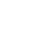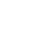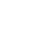# Ohm’s Law

The average person uses a lot of electricity in the many things they do every day. We are used to being able to turn on a light, watch the television, use our mobile devices, and stream music or videos. Because electricity is so much a part of our lives, we should know something about it.

Scientists create theories and laws to explain things that happen. In the 1800s, a German physicist named Georg Simon Ohm created Ohm’s Law to define the mathematical relationship between electric current, voltage, and resistance.

Ohm’s Law explains the way current flows through a resistance when a voltage (a different electric potential) is put on each end of the resistance.

An example might be that the voltage is the water pressure, the current is the amount of water that is going through a garden hose, and the resistance is the size of the garden hose.### The formula for Ohm’s Law

To make things easier, scientists create formulas and they give a kind of shortcut explanation. Ohm’s Law has a formula of:

V=IR

V=voltage, I=current, R=resistance

### Easier explanation of Ohm’s Law

A current is the free flowing of electrons through the conductors of a circuit. An electric circuit happens when a conductive path is made that lets the free electrons move continuously.

Voltage is the force that is motivating or moving the flow of electrons. Volts are a very specific measure of the potential energy that exists between the two points.

Resistance is anything that causes restriction of the movement of free electrons. When the free electrons move through conductors they contact some degree of opposition or friction, and this is called resistance.

To use Ohm’s Law for electric circuits you need to know at least two of the three values: either V, I, or R. When you know two of them, you can figure out the third. Ohm’s Law works in both the simple and complicated electric circuits and is considered to be a very important law.

### Finding the resistance:

The formula for finding the resistance is

R= V/I

Or resistance = volts divided by amps (current)

The formula for finding the amps is:

I = V/R

Or amps (current) = volts divided by resistance

Ohm’s Law indicates that the current that is passing through a conductor is proportional or comparable to the voltage over the resistance.

### Less Confusing:

Many of the scientific laws and principles can seem to be a bit confusing. One of the ways to remember Ohm’s Law is by using a triangle drawing.

### Application of Ohm’s Law in our Lives

When we read and study things such as Ohm’s Law we wonder exactly how will knowing this help us in our everyday live? But here’s the thing, once you know something like Ohm’s Law you can look around and see that it is in use in a lot of the things that surround us.

• Our heaters: Ohn’s Law is used in the heaters in our home to create heat. The conductor is designed to create a resistance to the free electron flow and that resistance gives us the heat that we need to keep warm.
• If you have a fan, you are witnessing Ohm’s Law. The speed of the fan is regulated using Ohm’s Law.
• The light from the bulbs in our lamps use Ohm’s Law.

## Fun Facts about Ohm’s Law:

• Ohm’s Law is usually not applied to AC (alternating current) because the current flow is constantly changing as well as other factors that can influence the flow of electricity.
• If you want to measure the volts in an electric circuit you can use a voltmeter.
• If you want to measure the amount of resistance in an electric circuit you can use an ohmmeter.
• A device that can measure quite a few functions including current, resistance, voltage, and temperature is a multimeter.

### Q&A

What does Ohm’s Law do?
defines the mathematical relationship between electric current, voltage, and resistance

What is the formula for Ohm’s Law?
V=IR

To use Ohm’s Law for electric circuits you need to know how many of the three values?
two

Of the two types of electricity, which one isn’t used by Ohm’s Law?
AC = alternating current

What is the device that professionals use to measure more than one function of the flow of electricity?
multimeter

•History
•Science
•Geography
•Biography
•US History